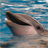Our World Statistics Day conversations have been a great reminder of how much statistics can inform our lives. Do you have an example of how statistics has made a difference in your life? Share your story with the Community!
Choose Language Hide Translation Barchuckp909
Staff (Retired)
Organizing Data for Optimal Analysis in JMP

One of the biggest challenges for Microsoft Excel users is modifying their Excel spreadsheets so the data can be properly analyzed in JMP. Below is an example of data an Excel user sent me:He asked how he could produce the above graph in JMP.

The majority of Excel users organize their time series data as shown above, with each column representing a period of time. And this makes sense to anyone who views such data in tabular form. But for JMP to graph this, the data needs to be reorganized. The JMP Graph Builder will not read multiple time periods that are broken up into separate columns as one continuous stream of data. It needs them organized into their common denominators. By that, I mean, the time variable (or dimension) needs to be one column.

So the first thing I did was use Tables->Stack to stack the time columns as shown below:Once I had the time values in one column, I was easily able to create the following graph:The one above duplicated the initial graph. I added the two below so you can compare the data by each day of the week. I prefer the trellis graph (the top one) because I can more easily compare each day’s calls from day to day.However, if you look at the original single line graph and compare it to the one I created in JMP, you’ll notice the JMP graph is not showing the average data point along with the line. If I ask JMP to display the dots as well, I’ll get the following:This is because, while JMP will calculate the average before it displays the line, it’s more informative to display all the data points in addition to the line than just the single calculated data point. The graph above not only shows the average value, but also how the data is spread for each time period. Notice how widely dispersed the calls are from 2:00-3:00.

Still I could reproduce the original graph by simply creating a new file with just the average amounts. First, I summarized the data using Table -> Summary:Then I used Graph Builder to graph from the following summarized table.Graphing this data and adding grid lines gives me a result that more closely resembles the original.Article Labels

There are no labels assigned to this post.

Article Tags